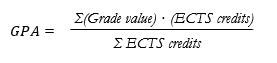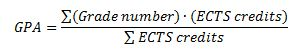# Grading at SSE - students enrolled from fall 2016

A new grading system at SSE is to be implemented for students enrolled from the Fall term of 2016. This concerns students at Bachelor and Master level.

1.5 credits for one full week of studies equal to 60 credits for a full academic year, and four passing grades: Excellent, Very Good, Good and Pass.

# GRADE POINT AVERAGE (GPA) - STUDENTS ENROLLED FROM FALL 2016

A Grade Point Average (GPA) is calculated on all approved courses graded on the Excellent – Pass grading scale. The calculation is performed by assigning a numerical value to each grade (Excellent = 5.00 points, Very Good = 4.00, Good = 3.50, Pass = 3.00) which is then weighted by the number of credits for each course. This is done by multiplying the numerical value by the number of credits for each course and then calculating the sum total. To calculate the GPA, the total is then divided by the total number of credits achieved as shown in the formula below:A student's GPA can vary between 3.0 and 5.0, where 5.0 is the highest. The GPA also includes any complementary courses taken beyond the degree requirement.

# ECTS GRADING AT SSE - STUDENTS ENROLLED BEFORE 2016

In 2007 the Stockholm School of Economics changed its grading system in conjunction with the transformation to the Bologna Model.

The main components of the ECTS grading system includes ECTS credits: 1.5 credits for one full week of studies equal to 60 credits for a full academic year, and five passing ECTS grades: A, B, C, D, E, and F for fail.

# GRADE POINT AVERAGE (GPA) - STUDENTS ENROLLED BEFORE 2016

A Grade Point Average (GPA) is calculated for all passed courses with a letter grade (A – E) in the following way: for each course the letter grade is converted to a numeric value using A = 5.00, B = 4.00, C = 3.00, D = 2.00 and E = 1.00. Then the values are weighted by the ECTS credits for each course. This is done by multiplying the value by the ECTS credits for each course and then calculating the sum of the products. This sum is then divided by the sum of the ECTS credits to give the GPA as shown in the formula:The GPA for a student can range from 1.00 - 5.00, with 5.00 being the highest. The GPA also includes any complementary courses taken beyond the degree requirement.

To access the grade performance of an SSE student, one can compare his/her letter grades and individual GPA to the Class GPA of all courses offered to the whole cohort, and to the distribution of grades of all courses offered during the entirety of the program (see below)

Den här hemsidan använder cookies. Genom att använda hemsidan accepterar du dessa cookies samt villkoren beskrivna i vår dataskyddspolicy. Läs mer